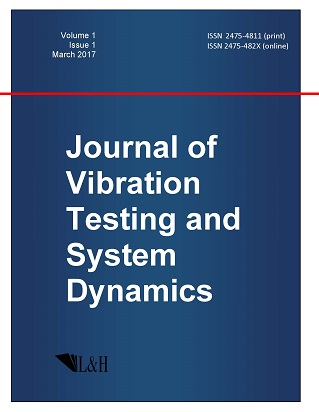ISSN: 2475-4811 (print)
ISSN: 2475-482X (online)
Journal of Vibration Testing and System Dynamics

C. Steve Suh (editor), Pawel Olejnik (editor),

Xianguo Tuo (editor)

Pawel Olejnik (editor)

Lodz University of Technology, Poland

Email: pawel.olejnik@p.lodz.pl

C. Steve Suh (editor)

Texas A&M University, USA

Email: ssuh@tamu.edu

Xiangguo Tuo (editor)

Sichuan University of Science and Engineering, China

Email: tuoxianguo@suse.edu.cn

A Generalized Lattice Boltzmann Model for Simulating Axisymmetric Convective Flow in Porous Media

Journal of Vibration Testing and System Dynamics 1(3) (2017) 207--217 | DOI:10.5890/JVTSD.2017.09.003

Zuo Wang , Jiazhong Zhang, Nannan Dang , Biwu Huang

School of Energy and Power Engineering, Xi’an Jiaotong University, Xi’an, Shaanxi 710049, PR China

Abstract

Axisymmetric convective flow in porous media is frequently encountered in nature and industry applications. In recent years, Lattice Boltzmann method has been developed as a powerful tool for such kind of flow and heat transfer. Despite its success in many problems regarding to porous flow, the existing LB model for the axisymmetric thermal flow in porous media at the representative volume scale suffers from a serious drawback. That is, it can not handle the cases where the heat capacitance of porous media varies spatially obviously. In this paper, a generalized LB model for axisymmetric temperature field is proposed to remedy this shortcoming. Chapman-Enskog analysis demonstrates that the energy equation in the cylindrical coordinates system can be recovered by the proposed model. Natural convection in a vertical annulus filled with saturated porous media, natural convection in a vertical annulus without porous media, have been carried out, and the results predicted by the present LB model agree well with the existing numerical data. More, natural convection in a vertical annulus with spatially varying heat capacitance shows that the present model can address the problem where the heat capacitance varies spatially obviously successfully.

Acknowledgments

This work was financially supported by the National Key Basic Research Program of China (973 Program) (No. 2012CB026002) and National Key Technology R&D Program of China (No. 2013BAF01B02).

References

1.   Vafai, K. (1984), Convective flow and heat transfer in variable-porosity media, Journal of Fluid Mechanics, 147, 233-259.
2.   Prasad, V. and Kulacki, F.A. (1985), Natural convection in porous media bounded by short concentric vertical cylinders, Journal of Heat Transfer, 107, 147-154.
3.   Prasad, V., Kulacki, F.A., and Keyhani, M. (1985) Natural convection in porous media, Journal of Fluid Mechanics, 150, 89-119.
4.   Vafai, K. (2005), Handbook of Porous Media, second ed., Taylor & Francis, New York.
5.   Nield, D.A. and Bejan, A. (2006), Convection in Porous Media, third ed., Springer, New York.
6.   Aidun, C.K. and Clausen, J.R. (2009), Lattice-Boltzmann Method for Complex Flows, Annual Review of Fluid Mechanics, 42, 439-472.
7.   He, Y.L., Wang, Y., and Li, Q. (2009), Lattice Boltzmann Method: Theory and Applications, Science Press, Beijing.
8.   Mohammad, A.A. (2011), Lattice Boltzmann method fundamentals and engineering applications with computer codes, SpringerVerlag.
9.   Xiang, X.Q., Wang, Z.H., and Shi, B.C. (2012), Modified lattice Boltzmann scheme for nonlinear convection diffusion equations, Communnications in Nonlinear Science and Numerical Simulatation, 17, 2415-2425.
10.   Li, Q., Luo, K.H., Kang, Q.J., He, Y.L., Chen, Q., and Liu, Q. (2015), Lattice Boltzmann methods for multiphase flow and phase-change heat transfer, Progress in Energy and Combustion Science, 52, 62-105.
11.   Zhang, J.Y. and Yan, G.W. (2016), Simulations of the fusion of necklace-ring pattern in the complex Ginzburg- Landau equation by lattice Boltzmann method, Communnications in Nonlinear Science and Numerical Simulatation, 33, 43-56.
12.   Rong, F.M., Guo, Z.L., Chai, Z.H., and Shi, B.C. (2010), A lattice Boltzmann model for axisymmetric thermal flows through porous media, International Journal of Heat and Mass Transfer, 53, 5519-5527.
13.   Guo, Z.L., Han, H.F., Shi, B.C., and Zheng, C.G. (2009), Theory of the lattice Boltzmann equation: lattice Boltzmann model for axisymmetric flows, Physical Review E, 79, 046708.
14.   Kods, G., Raoudha, C., Zied, L., Adel, B., Yves, B., and Abdelmajid, J. (2016), Lattice Boltzmann model for incompressible axisymmetric thermal flows through porous media, Physical Review E, 94, 043306.
15.   Halliday, I., Hammond, L.A., Care, C.M., Good, K., and Stevens, A. (2001), Lattice Boltzmann equation hydrodynamics, Physical Review E, 64, 011208.
16.   Li, Q., He, Y.L., Tang, G.H., and Tao, W.Q. (2009), Lattice Boltzmann model for axisymmetric thermal flows, Physical Review E, 80, 037702.
17.   Zheng, L., Shi, B.C., Guo, Z.L., and Zheng, C.G. (2010), Lattice Boltzmann equation for axisymmetric thermal flows, Computers and Fluids, 39, 945-952.
18.   Chen, S., Yang, B., and Zheng, C.G. (2017), A lattice Boltzmann model for heat transfer in porous media, International Journal of Heat and Mass Transfer, 111, 1019-1022.
19.   Guo, Z.L., Shi, B.C., and Zheng, C.G. (2002), Non-equilibrium extrapolation method for velocity and pressure boundary conditions in the lattice Boltzmann method, Chinese Physics B, 11, 366-374.
20.   Li, L., Mei, R., and Klausner, J.F. (2013), Multiple-relaxation-time lattice Boltzmann model for the axisymmetric convection diffusion equation, International Journal of Heat and Mass Transfer, 67, 338-351.